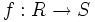# Integral morphism

Suppose$R$ and$S$ are commutative unital rings and$f:R \to S$ is a homomorphism of commutative unital rings. Then, we say that$f$ is an integral morphism if$S$ is an integral extension of the image of$R$ in$S$. Equivalently, we say that$f$ is an integral morphism if every element of$S$ satisfies a monic polynomial with coefficients in$R$.